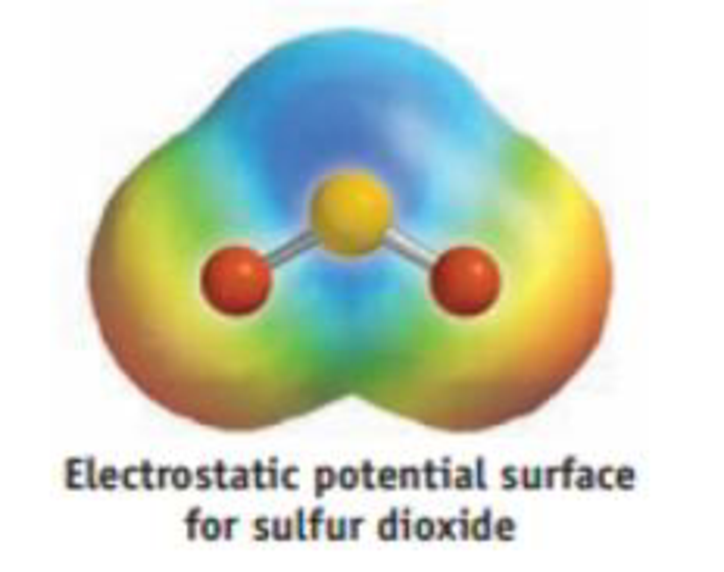Chapter 8, Problem 66GQ

Chapter
Section
Textbook Problem

Draw resonance structures for the SO2 molecule, and determine the formal charges on the S and O atoms. Are the S—O bonds polar, and is the molecule as a whole polar? If so, what is the direction of the net dipole in SO2? Is your prediction confirmed by the electrostatic potential surface? Explain briefly.Interpretation Introduction

Interpretation: The resonance structure for SO2 molecule should be determined with formal charge on S atom and O atoms. The molecule and bond S-O is polar or not should be identified and the direction of net dipole should be indicated.

Concept Introduction:

Electronegativity: It is defined as the capacity of the atom to abstract the pair of electrons towards itself results to have high negative charge.

Polar molecule: The molecule with atoms bonded with different electronegativity. Dipole moment is used to measure the polarity of the molecule.

Polarity of a molecule is measured in term of dipole moment.

Dipole moment for a polar molecule is non-zero and for a non-polar molecule dipole moment is zero.

Electrostatic potential map: It is 3D representation of molecules which shows the distribution of charges present in the molecule. Generally red, orange, yellow, green and blue defines the charge potentials present in the molecule.

RED<ORANGE<YELLOW<GREEN<BLUE

The blue color indicates the most positive potential end and the red color defines the most negative potential end.

Explanation

The Lewis structure for the given following molecule is determined as below,

The Lewis structure of the SO2 molecules identified as follows,

The Lewis electron dot structure for given molecules are determined by first drawing the skeletal structure for the given molecules, then the total number of valence electrons for all atoms present in the molecules are determined.

The next step is to subtract the electrons present in the total number of bonds present in the skeletal structure of the molecule with the total valence electrons such that considering each bond contains two electrons with it.

Finally, the electrons which got after subtractions have to be equally distributed considering each atom contains eight electrons in its valence shell.

The Resonance structure for the given molecule is shown by moving the lone pair and pi electrons as follows,

Still sussing out bartleby?

Check out a sample textbook solution.

See a sample solution

The Solution to Your Study Problems

Bartleby provides explanations to thousands of textbook problems written by our experts, many with advanced degrees!

Get Started

Find more solutions based on key concepts